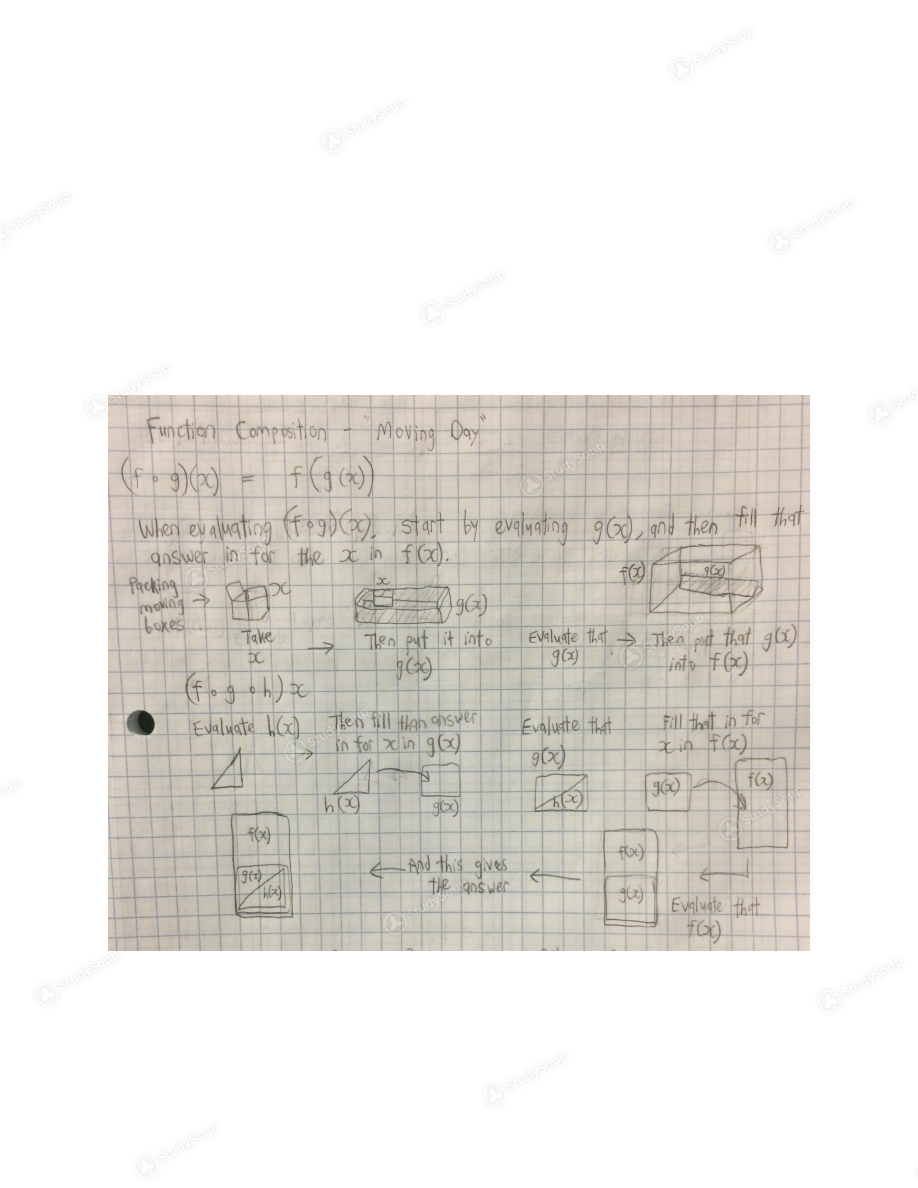Limited time offer 20% OFF StudySoup Subscription details

# Clemson - MATH 1040 - Study Guide - Final

### Created by: cmmarr Elite Notetaker

> > > > Clemson - MATH 1040 - Study Guide - Final

Clemson - MATH 1040 - Study Guide - Final

5 5 3 27 Reviews
This preview shows pages 1 - 4 of a 13 page document. to view the rest of the contentStudy Guide for Math 1040 Final Exam
A Compilation of Study Guides for Tests 1, 2, and 3, and the Material Covered
Afterward
Relations and Functions, Testing for Functions, Domain and Range A relation is what happens to an input to convert it into an output.
X is the independent variable – what is filled into the function – and Y is the
dependent variable – the results that you obtain.
A function is a special kind of relation, in which each input corresponds to exactly
one output.
If you fill in a particular number for x, you will always obtain the same y-value for
that x. If one x value gives you two different possible y values, then it is not a
function.
Testing to See If a Relation is a Function: Analytical Method – plug many number
values in for x and evaluate. If each x yields one and only one y-value, then it is a
function.
Graphical Method – Graph the function in the x-y plane. If you draw a vertical line
anywhere over the relation and that line crosses the relation only once, then it is a
function. This is called the Vertical Line Test.
The outputs of a function must always be classified as real numbers. So, some
functions have restrictions about what inputs can be put in for x, to ensure that the
outputs stay real.
Domain: The set of allowable inputs for a function.
Range: The set of allowable outputs for a function.
Situations to Avoid in Domain and Range:
The domain and range are essentially all real numbers, excluding those that would
yield these three situations when filled in for a function:
1. Division by zero.
2. The square root of a negative number – or any even root of a negative
number. 3. The log 10  of a negative number. Writing Domain and Range:
Domain and Range are stated using brackets or parentheses.
Use ( ) if the domain does not include the number at the end.
Use [ ] if the domain does include the number at the end.
The union symbol U lies between two parenthetical expressions to indicate
everything in either parentheses.
The intersection symbol
between two parenthetical expressions indicates only  items that lie in both expressions. Composition of FunctionsIf f and g are functions, then all these are also functions. (f+g)(x) = f(x) + g(x) (f-g)(x) = f(x) + g(x) (f * g)(x) = f(x) * g(x) (f/g) (x) = f(x) / g(x) When combining functions, if one of the functions has a smaller domain than the
other, the domain of the new, combined function must shrink to the smaller domain
– the “weak link,” of the combination.
Transformations of Functions  Y changes:
f(x) + h
moves f(x) up in the y-axis by h units f(x) – h  moves f(x) down in the y-axis by h unitsX changes:
f(x+h)
moves f(x) h units to the left f(x-h)  moves f(x) h units to the right Length (Vertical Expansion/Contraction):
h*f(x)
“Heightens” f(x) by a factor of h (1/h) f(x) “Shortens” f(x) by a factor of h Width (Horizontal Expansion/Contraction):
F(xh)
“Compresses” f(x) by a factor of h F(x*1/h) “Stretches” f(x) by a factor of h Rule of thumb:
In the y plan, operations to functions perform their expected effects, and they go in
the order of operations.
In the x plane, operations to functions have the opposite effect of their operation,
and they go in the reverse order of operations.
Polynomials  Polynomials are functions of this form:

a 0  + a x +a x  2  + a x 3  …a n-1  x n-1  + a n  x n The largest power in the polynomial is the polynomial’s degree. Various Degrees of Polynomials:
Line: y = mx + b
2  + bx + c Cubic: a 1  x 3  + a 2 x + a 3 x + a 4 All degrees beyond cubic are simply called polynomials. Lines: All you need to graph a line is either two points, or one point and a slope.
If a line passes through two points, (x
1 , y 1 ) and (x 2 , y 2 ), then the slope m of the line  can be found: M = y 2  – y Or  y - y 2          X 2  – x x 1  – x 2 To find the y-intercept for a line: Plug in 0 for x and solve for y.
Y-intercept Form of a Line: y = mx + b
Point Slope Form of a Line: (y
1 -y 2 ) = m (x – x 2 ) To find a line parallel to another line: Lines with the same slope are always parallel.
To find a line parallel to another and construct it passing through a certain point,
simply fill the slope and that point into the y-intercept form and it will give you the
y-intercept and hence the equation.
To find a line perpendicular to another line: Take the negative inverse of the slope. If
the original slope is m, then the new slope will be – 1/m. If you are looking for a
perpendicular line that passes through a particular point, fill the new slope and thatpoint into the y-intercept form of a line and that will give you the new line’s
equation.
Quadratics: ax + bx +c Domains of polynomials generally encompass all real numbers. Some, however,
have restrictions based on the Forbidden Three.
A root of a polynomial p(x) is an x that makes px equal 0. These are also called x
intercepts, zeros, or solutions.
The Fundamental Theorem of Algebra:
X= a is a root of a polynomial p(x)
If and only if
There is a polynomial a(x) such that px = (x-a) a(x).
In other words, if a is a number, and if you can factor out (x-a) from a polynomial,
then whatever a is can be substituted in for x and it will make the polynomial equal
0.
Basic principle: If a is a root, then x – a is a factor.
One thing to keep in mind: you can factor a polynomial and this will give you all its
roots, but it will not necessarily give you all its possible factors.
Methods of solving quadratics for roots, in the general order that they should be
used:
Factoring
Completing the square
The Rational Root Test
Factoring:
When solving for the roots of the polynomial ax
2 +bx+c, first try factoring. If the polynomial’s leading coefficient is 1: 1. Set the polynomial equal to zero.
2. Try to find two numbers that will multiply together to equal c and add to
equal b.  3. Use these two numbers (let’s call them p and q) to form factors of the  polynomial: (x-p) and (x-q). If p or q is positive, then they are subtracted from
x. If they are negative, they are added.
When factoring a polynomial with a leading coefficient greater than 1, you can
either:
1. Find two numbers that multiply to equal a and add to equal b – or
2. Factor out the leading coefficient first and then factor the rest of the
polynomial. Difference of Squares Rule:
X
2 -a 2  = (x-a) (x+a) Factoring Cubics:
X
3 -a 3  = (x-a) (x 2 + ax + a 2 ) X 3 +a 3  = (x +a) (x 2  – ax + a 2 ) Higher Orders:

This is the end of the preview. Please to view the rest of the contentJoin more than 18,000+ college students at Clemson University who use StudySoup to get ahead
##### Description: A compilation of all the material in the study guides for tests 1, 2, and 3, and a brief summary of the material covered since then (derivatives of logarithmic functions).
13 Pages 31 Views 24 Unlocks
• Notes, Study Guides, Flashcards + More!
Get Full Access to Clemson - MATH 1040 - Study Guide - Final
×
Get Full Access to Clemson - MATH 1040 - Study Guide - Final

I don't want to reset my password

Need help? Contact support

Need an Account? Is not associated with an account
We're here to help